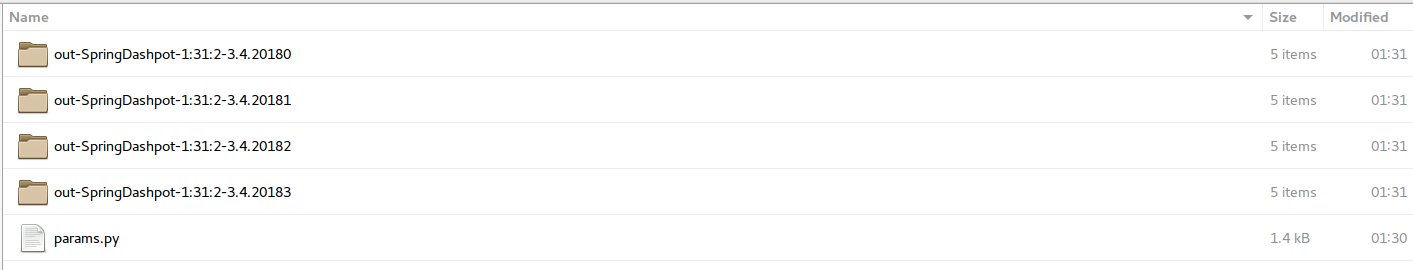# Single script multiple parameter:¶

This is a sample script that demonstrated pygran's "single script multiple parameter" feature. This enables users to do sensitivity analysis and parameterization studies using the same input script. In this example, the coefficient of restitution is varied between 0.225, 0.3, 0.45, and 0.9 for a viscoelastic model (spring-dashpot). 4 different simulations are run simultaneously in parallel, each corresponding to a unique coefficient of restitution. The input script and mesh files are found here.

# Stage 1: data structure creation¶

First, a python dictionary (params) defining the simulation parameters is created as shown below. The parameter 'nSim' defines the number of simulations to run, must correspond to the number of parameter values to be varied (in this case coefficient of restitution).

In [ ]:
# Import the analysis module and an organic powder (template)
from pygran import simulation
from pygran.params import organic

# Launch 4 simultaneous simulations, each with a different coef of rest
nSim = 4
materials = [organic.copy() for i in range(nSim)]

for i, mat in enumerate(materials):
mat['coefficientRestitution'] = 0.9 / (1 + i)

# Create a dictionary of physical parameters
params = {

# Define the system
'boundary': ('p','p','f'), # fixed BCs
'box':  (-0.001, 0.001, -0.001, 0.001, 0, 0.004), # simulation box size

# Define component(s), materials is a list of dictionary of size nSim
'species': ({'material': materials, 'radius': ('constant', 2e-4)},
),

# Timestep
'dt': 2e-6,

# Apply gravitional force in the negative direction along the z-axis
'gravity': (9.81, 0, 0, -1),

# Number of simulation steps (non-pygran variable)
'nsteps': 1e3,

# number of concurrent simulations to run
'nSim': nSim
}


# Stage 2: simulation¶

The params dictionary is then used to create a pygran.DEM class and run the simulation.

In [ ]:
# Create an instance of the DEM class
sim = simulation.DEM(**params)

# Setup a primitive wall along the xoy plane at z=0
sim.setupWall(species=1, wtype='primitive', plane = 'zplane', peq = 0.0)

# Insert the particles
insert = sim.insert(species=1, value=100, region=('block', -1e-3,1e-3, -1e-3, 1e-3, 0, 3e-3))
sim.run(params['nsteps'], params['dt'])
sim.remove(insert)

# Relax the system
sim.run(params['nsteps'], params['dt'])


# Output¶

The output is a set of 4 folders created in the working directory, each containing output files (traj and restart files) corresponding to a unique coefficient of restitution. A snapshot of the 4 folders is shown below.

In :
from IPython.display import Image
Image(filename='folders.png', width=1200, height=1600)

Out: